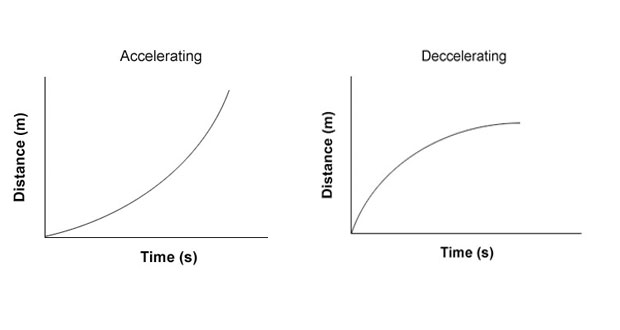# Graphs Of Motion & BiomechanicsGraphs are a good way of showing motion visually. The two types of graphs which are of most interest are distance/time graphs and speed/time (or velocity/time) graphs. Graphs are particularly useful when things are moving too fast for the naked eye and brain to understand what is happening, which is often the case in sport.

## Distance/time graphs

Time is always plotted along the bottom (x-axis) and distance is always up the side (y-axis). The graph below shows a body that is stationary (not moving at all). As time passes the distance does not change… simple! Think of a sporting example other than a racing car sat on the start line.

The graph below shows a body moving at constant velocity. As time passes the distance increased uniformly. Note the gradient of this graph is equal to the speed of the body (speed – distance/time). So in this example speed = 2m / 10s = 0.2 m/s. Think of an example in sport other than the racing car cruising at a constant speed on the home straight having passed the chequered flag?

The graph below shows a body accelerating. The change in distance increases and time passes so it must be getting faster and faster.

The graph below shows a body decelerating. The change in distance decreases as time passes so it must be slowing down.

## Velocity/time  graphs

A velocity against time graph shows how fast a body was going at a particular instant in time. Time is always plotted on the bottom (X-axis) and velocity is up the side (Y-axis). This could be useful for coaching analysing a 100m sprint where the velocity of the athlete will change throughout the race.

The graph below shows velocity is not changing as time passes (so the body is moving at a constant velocity)

The graph below shows a body accelerating, for example, a sprinter during the first 20m of a 100m race.

The graph below shows a body decelerating, for example, the same sprinter during the last 20m of a 100m sprint.